# Lines

How many lines can be draw with 8 points, if three points lie on one line and the other any three points do not lie on the same line?

n =  26

### Step-by-step explanation:

$n=1+3+4+5+6+7=26$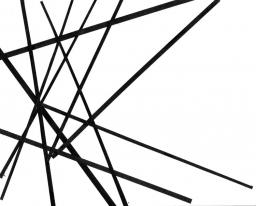Did you find an error or inaccuracy? Feel free to write us. Thank you!Tips to related online calculators
Would you like to compute count of combinations?

## Related math problems and questions:

• Points in space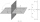There are n points, of which no three lie on one line and no four lies on one plane. How many planes can be guided by these points? How many planes are there if there are five times more than the given points?
• LinesIn how many points will intersect 14 different lines, where no two are parallel?
• Points in planeThe plane is given 12 points, 5 of which is located on a straight line. How many different lines could by draw from this points?
• CirclesHow many different circles are determined by 9 points at the plane if 6 of them lie in a straight line?
• Three points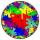Mark three points E, F and G in the plane not lie on one line. a) Draw a line segment FG b) Construct halfline (ray) EG c) Draw a line EF
• Elections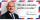In elections candidate 10 political parties. Calculate how many possible ways can the elections finish, if any two parties will not get the same number of votes.
• Parallels and one secant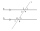There are two different parallel lines a, b, and a line c, that intersect the two parallel lines. Draw a circle that touches all lines at the same time.
• Combinations of sweatersI have 4 sweaters two are white, 1 red and 1 green. How many ways can this done?
• The camp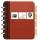At the end of the camp a 8 friends exchanged addresses. Any friend gave remaining 7 friends his card. How many addresses they exchanged?
• Combi-triangle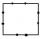On each side of the square is marked 10 different points outside the vertices of the square. How many triangles can be constructed from this set of points, where each vertex of the triangle lie on the other side of the square?
• PrizeHow many ways can be rewarded 9 participants with the first, second, and third prizes in a sports competition?
• Lie/do not lie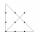The function is given by the rule f(x) = 8x+16. Determine whether point D[-1; 8] lies on this function. Solve graphically or numerically and give reasons for the your answer.
• Three-digit numbersHow many three-digit numbers are from the numbers 0 2 4 6 8 (with/without repetition)?
• Subsets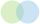How many 19 element's subsets can be made from the 26 element set?
• Phone numbersHow many 7-digit telephone numbers can be compiled from the digits 0,1,2,..,8,9 that no digit is repeated?
• Tournament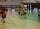Determine how many ways can be chosen two representatives from 34 students to school tournament.
• Sweater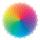Dana confuses sweater and wool has a choice of seven colors. In how many ways can she choose from three colors to the sleeves?